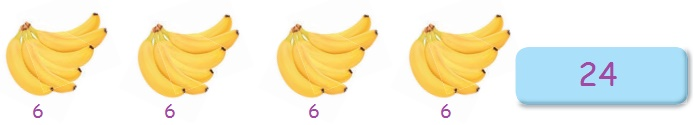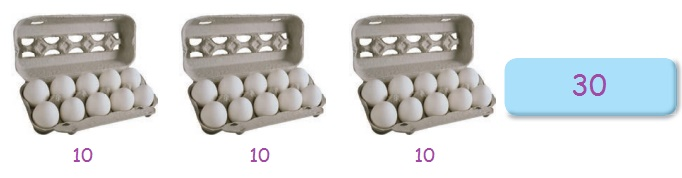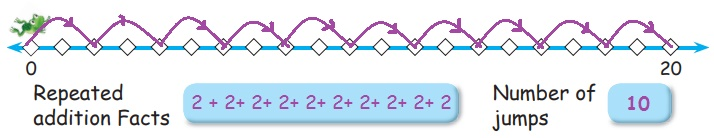Home | | Maths 2nd Std | Repeated Addition

## Chapter: 2nd Maths : Term 3 Unit 1 : Numbers

Teacher’s Note: Teacher shall narrate the situation to explain the concept of repeated addition.

Travel Through

Footwear Shop

Mugilan and Yazhini went to a footwear shop. By the time they entered the shop they saw a few of their friends buying footwear. To their surprise, they found the shop was left empty after sometime.So, they returned home without buying footwear. Can you say, why?

Read the following conversation. Mugilan and Yazhini explained the situation to their mother.

Yazhini : Mom, I saw chitu, She flew away with 2 sets of 2 slippers ( 2 + 2 = 4)

Mother : Oh! I see.

Mugilan : I saw pussyin the shop. It went away with 3 sets of 4 slippers (4 + 4 + 4 = 12)

Mother : Is it so?

Yazhini : Mom! The flyselected 4 sets of 6 shoes. (6+6+6+6= 24).

Mother : What happened next?

Mugilan : Spiderchose 5 sets of 8 shoes.

(8+8+8+8+8=40) If none of the slippers was left in the shop. Can you guess who came next ?

Yazhini : Finally Centipedecame and emptied the shop.

Teacher’s Note: Teacher shall narrate the situation to explain the concept of repeated addition.

Learn

Vegetable farm

Agilan and Azhagi are interested in gardening. In the leisure time, they are always found in the vegetable garden in the backyard. They have four types of vegetable plants. Let us visit the garden.Teacher’s Note: Teacher can create different situation for repeated addition and make children practice in class.

Practice

Use repeated addition to find the total.

1. There are 5 groups. There are 3 chicks in each group. How many chicks are there altogether?Asn : 3 + 3 + 3 + 3 + 3 = 15 chicks

2. There are 4 bunches. There are 6 bananas in each bunch. How many bananas are there altogether?Ans : 6 + 6 + 6 + 6 = 24 bananas

3. There are 3 trays. There are 10 eggs in each tray. How many eggs are there altogether?Ans : 10 + 10 + 10 = 30 eggs

Teacher’s Note: Teacher can make children observe that repeated addition of even numbers is even but repeated addition of odd numbers is either even or odd.

Pleasure Time

Draw the missing figures and complete repeated addition fact.Ans:

ii) 2 + 2 + 2 + 2 = 8

iii) 5 + 5 + 5 = 15

iv) 6 + 6 + 6 + 6 = 24

Learn

Let us learn repeated addition using number line.Start from 0 and leap in three’s upto 12.

Frog will reach 12 in 4 leaps of 3 units each.

The frog can leap 3 units distance in one attempt.

After the 4th attempt the distance covered by the frog will be 12 units it is shown as 0 + 3 = 3; 3 + 3 = 6; 6 + 3 = 9; 9 + 3 =12 that is 3 when repeatedly added 4 times gives 12 which can be expressed as 3 + 3 + 3 + 3 = 12 units.

Practice

For each of the distances given below, draw the leaping positions of the frog on the line to reach 20.

i) one leap covers 2 units distance.Repeated addition facts  2 + 2+ 2+ 2+ 2+ 2+ 2+ 2+ 2+ 2 Number of jumbs 10

ii) one leap covers 4 units distance.Repeated addition facts 4 + 4 + 4 + 4 + 4  Number of jumbs 5

iii) one leap covers 5 units distance.Repeated addition facts 5 + 5 + 5 + 5 Number of jumbs 4

Mental Maths

1. Aditya bought 6 packets of groundnut balls. Each packet had 5 groundnut balls. How many groundnut balls did he buy?Ans : 5 + 5 + 5 + 5 + 5 + 5 = 30

2. There are 10 boats. Each boat can accomodate 5 children in it. How many children are there in all?Ans : 5 + 5 + 5 + 5 + 5 + 5 + 5 + 5 + 5 + 5 = 50

3. Ranjith had a bag that can hold 5 note books. If he had 6 such bags then how many note books does he have in total?Ans : 5 + 5 + 5 + 5 + 5 + 5 = 30

4. Maghizhini saves 10 rupees a day. How much will she save in 7 days?Ans : 10 + 10 + 10 + 10 + 10 + 10 + 10 = 70

5. There are 7 plates. Each of the plates has 3 fruits in it. How many fruits are there in all?Ans : 3 + 3 + 3 + 3 + 3 + 3 + 3 = 21

Tags : Numbers | Term 3 Chapter 1 | 2nd Maths , 2nd Maths : Term 3 Unit 1 : Numbers
Study Material, Lecturing Notes, Assignment, Reference, Wiki description explanation, brief detail
2nd Maths : Term 3 Unit 1 : Numbers : Repeated Addition | Numbers | Term 3 Chapter 1 | 2nd Maths